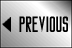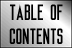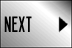STAR FLEET BATTLES ERRATA

## Star Fleet Battles Errata: (R5.0)

(R5.F2) Most Kzinti SSDs have TADS fighters.

(R5.F8) Kzinti LAS does indeed have 2 special and 2 standard rails (plus 4 light rails). The SSD should reflect this.

(R5.PF2) A non-SCS PFT carrying a single MRN would have one of each type of pallet, although this would have been a very unusual situation since there were so few MRNs, and would reflect that an SCS had been lost in combat. Non-SCS PFTs cannot purchase more than one MRN.

(R5.R1) These ships received DERFACS in Y168. There is no cost reduction prior to that time.

(R5.R5) D-racks on bases are also not covered by this refit.

(R5.02) CS SSD: RS = RF + R + RR (not + RF).

(R5.03) BC SSD: RS = RF + R + RR (not + RF). Drone racks should be 1+3 on left and 2+4 on right.

(R5.05) CL SSD: RS = RF + R + RR (not + RF).

(R5.08) FF SSD: RS = RF + R + RR (not + RF).

(R5.18) SF SSD: RS = RF + R + RR (not + RF).

(R5.20) EFF SSD: Has two deck crews, not one.

(R5.21) MS SSD: RS = RF + R + RR (not + RF).

(R5.22) PFT SSD: Can control a number of seeking weapons equal to the sensor rating.

(R5.23) DF SSD: RS = RF + R + RR (not + RF). The second drone rack #3 and #4 should be #5 and #6.

(R5.28) MEC: There are more counters in Module R2.

(R5.36) POL SSD: RS = RF + R + RR (not + RF).

(R5.37) SR SSD: RS = RF + R + RR (not + RF). The C14 refit began in Y170.

(R5.41) FH SSD: RS = RF + R + RR (not + RF).

(R5.46) FFK SSD: RS = RF + R + RR (not + RF).

(R5.47) CD SSD: RS = RF + R + RR (not + RF).

(R5.48) CA SSD: RS = RF + R + RR (not + RF).

(R5.53) The ADD was increased to 12 rounds by the Y175 refit, prior that it had six rounds.

(R5.55) SDF SSD: RS = RF + R + RR (not + RF).

(R5.59) Kzinti BCV should have range-40 disruptors.

(R5.60) Kzinti BCS should have range-40 disruptors.